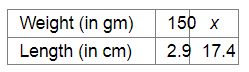# The amount of extension in an elastic string varies directly as the weight hung on it.`
Question:

The amount of extension in an elastic string varies directly as the weight hung on it. If a weight of 150 gm produces an extension of 2.9 cm, then what weight would produce an extension of 17.4 cm?

Solution:

Let x gm be the weight that would produce an extension of 17.4 cm.Since the amount of extension in an elastic string and the weight hung on it are in direct variation, we have:

$\frac{150}{x}=\frac{2.9}{17.4}$

$\Rightarrow 17.4 \times 150=2.9 \times x$

$\Rightarrow x=\frac{17.4 \times 150}{2.9}$

$=\frac{2610}{2.9}$

$=900$

Thus, the required weight will be $900 \mathrm{gm}$.